## Machine Learning

A collection of articles on Machine Learning that teach you the concepts and how they’re implemented in practice.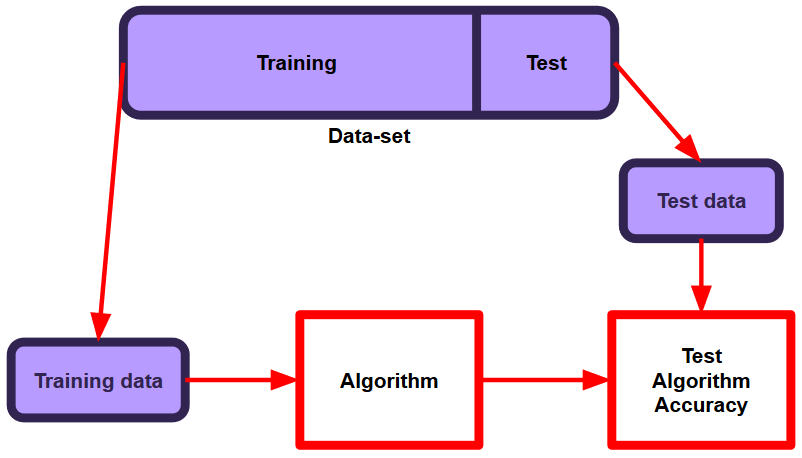## Overview

This will be the third article in a four-part series covering the following:

1. Dataset analysis - We will present and discuss a dataset selected for our machine learning experiment. This will include some analysis and visualisations to give us a better understanding of what we're dealing with.

2. Experimental design - Before we conduct our experiment, we need to have a clear idea of what we're doing. It's important to know what we're looking for, how we're going to use our dataset, what algorithms we will be employing, and how we will determine whether the performance of our approach is successful.

3. Implementation - We will use the Keras API on top of TensorFlow to implement our experiment. All code will be in Python, and at the time of publishing everything is guaranteed to work within a Kaggle Notebook.

4. Results - Supported by figures and statistics, we will have a look at how our solution performed and discuss anything interesting about the results.

## Implementation

In the last article we covered a number of experimental design issues and made some decisions for our experiments. We decided to compare the performance of two simple artificial neural networks on the Iris Flower dataset. The first neural network will be the control arm, and it will consist of a single hidden layer of four neurons. The second neural network will be the experimental arm, and it will consist of a single hidden layer of five neurons. We will train both of these using default configurations supplied by the Keras library and collect thirty accuracy samples per arm. We will then apply the Wilcoxon Rank Sums test to test the significance of our results.A simple training and testing strategy.

With our dataset analysis and experimental design complete, let's jump straight into coding up the experiments.

If your desired dataset is hosted on Kaggle, as it is with the Iris Flower Dataset, you can spin up a Kaggle Notebook easily through the web interface: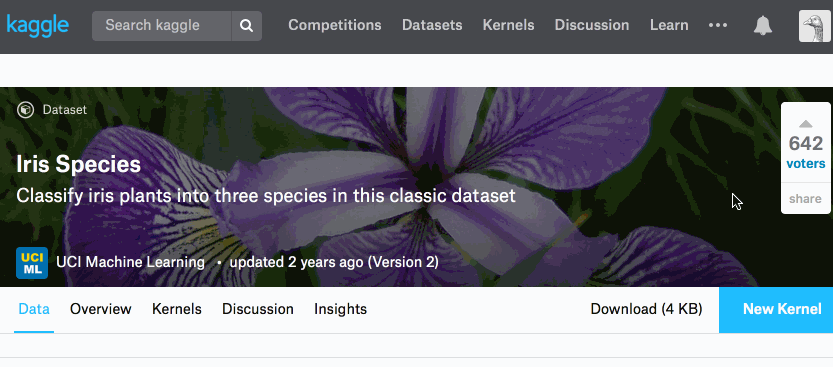Creating a Kaggle Notebook with the Iris dataset ready for use.

You're also welcome to use your own development environment, provided you can load the Iris Flower dataset.

## Import packages

Before we can make use of the many libraries available for Python, we need to import them into our notebook. We're going to need numpy , pandas , tensorflow , keras , and sklearn. Depending on your development environment these may already be installed and ready for importing. You 'll need to install them if that's not the case.

import numpy as np # linear algebra
import pandas as pd # data processing, CSV file I/O (e.g. pd.read_csv)

import tensorflow as tf # dataflow programming
from tensorflow import keras # neural networks API
from sklearn.model_selection import train_test_split # dataset splitting


If you're using a Kaggle Kernel notebook you can just update the default cell. Below you can see I've included imports for tensorflow , keras , and sklearn.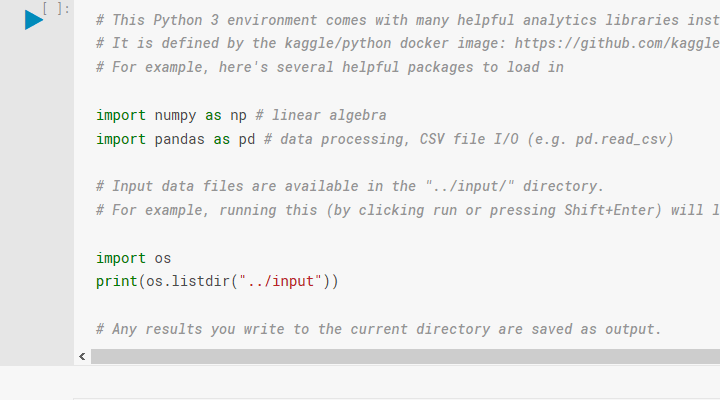To support those using their own coding environment, I have listed the version numbers for the imported packages below:

• tensorflow==1.11.0rc1
• scikit-learn==0.19.1
• pandas==0.23.4
• numpy==1.15.2

## Preparing the dataset

First, we load the Iris Flower dataset into a pandas DataFrame using the following code:

# Load iris dataset into dataframe


### Input parameters

Now, we need to separate the four input parameters from the classificatio labels. There are multiple ways to do this, but we're going to us pandas.DataFrame.iloc, which allows selection fro the DataFrame using integer indexing.

# Splitting data into training and test set
X = iris_data.iloc[:,1:5].values


With the above code we have selected all the rows (indicated by the colon) and the columns at index 1, 2, 3, and 4 (indicated by the 1:5). You may be wondering why the fifth column was not included, as we specified 1:5, that's because in Python we're counting from one up to five, but not including it. If we wanted the fifth column, we'd need to specify 1:6. It's important to remember that Python's indexing starts at 0, not 1. If we had specified 0:5, we would also be selecting the "Id" column.

To remind ourselves of what columns are at index 1, 2, 3, and 4, let's use the pandas.DataFrame.head method from the first part.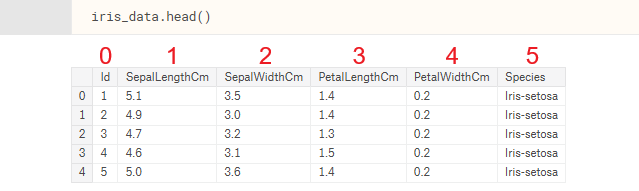Samples from the Iris Flower dataset with the column indices labelled in red.

We can also print out the contents of our new variable, X, which is storing all the Sepal Length/Width and Petal Length/Width data for our 150 samples. This is all of our input data.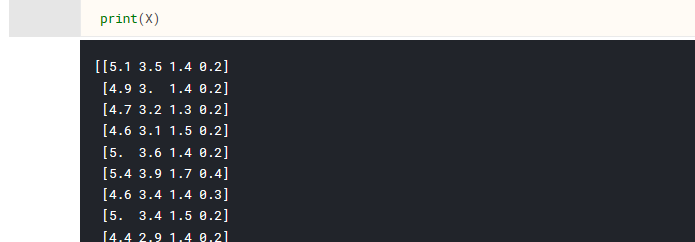The input data selected from the Iris Flower dataset.

For now, that is all the processing needed for the input parameters.

### Classification labels

We know from our dataset analysis in part 1 that our samples are classified into three categories, " Iris-setosa ", " Iris-virginica ", and " Iris- versicolor ". However, this alphanumeric representation of the labels is not compatible with our machine learning functions, so we need to convert them into something numeric.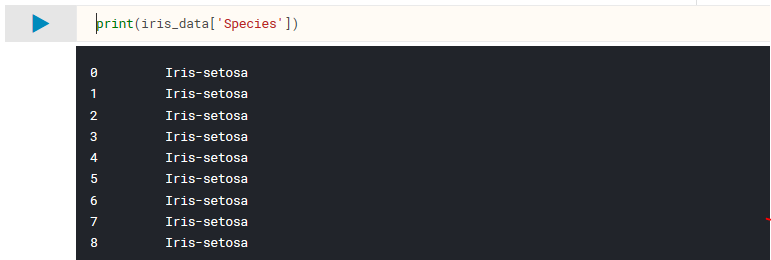Again, there are many ways to achieve a similar result, but let's use pandas features for categorical data. By explicitly selecting the Species column from our dataset as being of the category datatype, we can use pandas.Series.cat.codes to get numeric values for our class labels.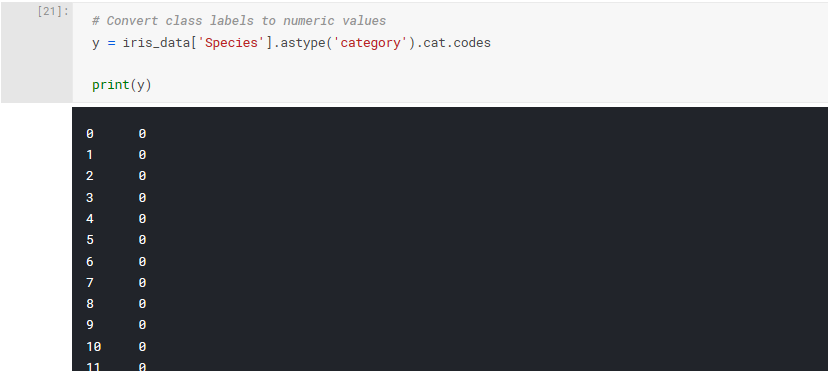We have one extra step, because we plan on using the categorical_crossentropy objective function to train our model. The Keras documentation gives the following instructions:

When using the categorical_crossentropy loss, your targets should be in categorical format (e.g. if you have 10 classes, the target for each sample should be a 10-dimensional vector that is all-zeros except for a 1 at the index corresponding to the class of the sample).

Keras Documentation (https://keras.io/losses)

What this means is we will need to use One-hot encoding. This is quite typical for categorical data which is to be used with machine learning algorithms. Here is an example of One-hot encoding using the Iris Flower dataset: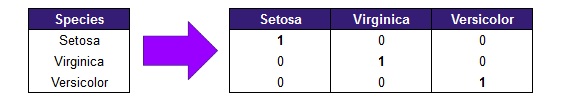One-hot encoding of the Iris Flower dataset class labels.

You can see that each classification label has its own column, so Setosa is $1,0,0$, Virginica is $0,1,0$, and Versicolor is $0,0,1$.

Luckily encoding our labels using Python and Keras is easy, and we've already completed the first step which is converting our alphanumeric classes to numeric ones. To convert to One-hot encoding we can use keras.utils.to_categorical():

# Use One-hot encoding for class labels
Y = keras.utils.to_categorical(y,num_classes=None)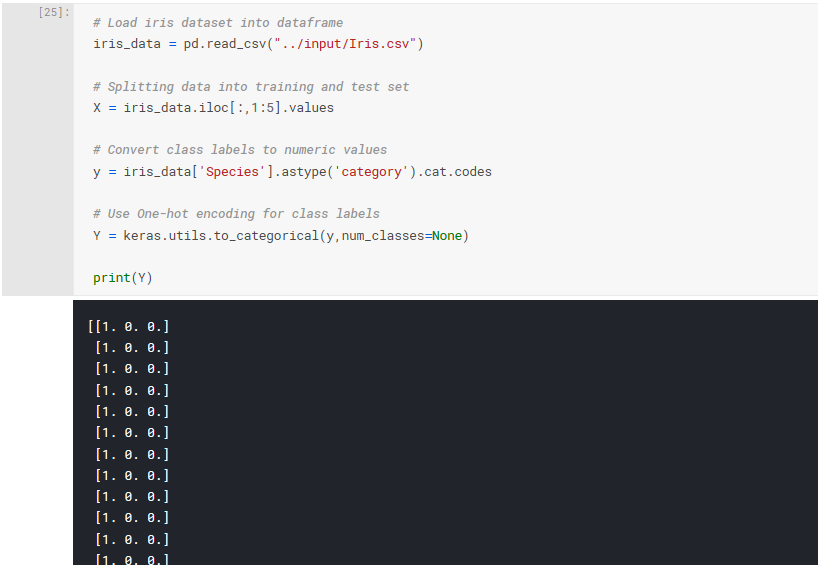### Training and testing split

In the previous part of this series we decided on the following:

The Iris Flower dataset is relatively small at exactly 150 samples. Because of this, we will use 70% of the dataset for training, and the remaining 30% for testing, otherwise our test set will be a little on the small side.

Machine Learning with Kaggle Notebooks - Part 2

This is where sklearn.model_selection.train_test_split() comes in. This function will split our dataset into a randomised training and testing subset:

# split into randomised training and testing subset
X_train, X_test, y_train, y_test = train_test_split(X,Y,test_size=0.3,random_state=0)


This code splits the data, giving 30% (45 samples) to the testing set and the remaining 70% (105 samples) for the training set. The 30/70 split is defined using test_size=0.3 and random_state=0 defines the seed for the randomisation of the subsets.

These have been spread across four new arrays storing the following data:

• X_train : the input parameters, to be used for training.

• y_train : the classification labels corresponding to the X_train above, to be used for training.

• X_test : the input parameters, to be used for testing.

• y_test : the classification labels corresponding to the X_test above, to be used for testing.

Before moving on, I recommend you have a closer look at the above four variables, so that you understand the division of the dataset.

## Neural networks with Keras

Keras is the software library we will be using through Python, to code up and conduct our experiments. It's a user friendly high-level neural networks library which in our case will be running on top of TensorFlow. What is most attractive about Keras is how quickly you can go from your design to the result.

### Configuring the model

The keras.Sequential() model allows you to build a neural network by stacking layers. You can add layers using the add() method, which in our case will be Dense() layers. A dense layer is a layer in which every neuron is connected to every neuron in the next layer. Dense() expects a number of parameters, e.g. the number of neurons to be on the layer, the activation function, the input_shape (if it is the first layer in the model), etc.

model = keras.Sequential()



In the above code we have created our empty model and then added two layers, the first is a hidden layer consisting of four neurons which are expecting four inputs. The second layer is the output layer consisting of our three output neurons.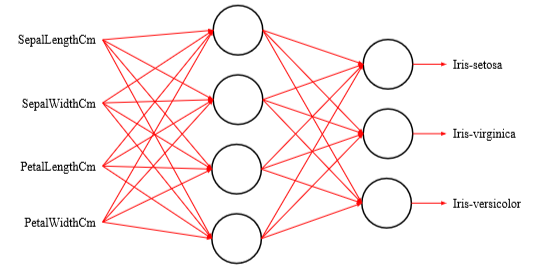We then need to configure our model for training, which is achieved using the compile() method. Here we will specify our optimiser to be Adam(), configure for categorical classification, and specify our use of accuracy for the metric.

model.compile(keras.optimizers.Adam(), 'categorical_crossentropy', metrics=['accuracy'])


At this point, you may wish to use the summary() method to confirm you've built the model as intended: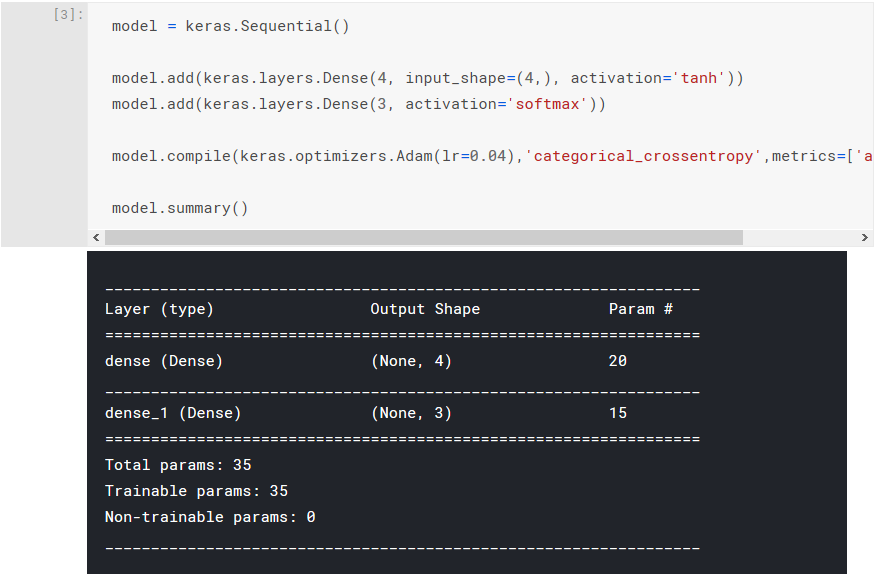### Training the model

Now comes the actual training of the model! We're going to use the fit() method of the model and specify the training input data and desired labels, the number of epochs (the number of times the training algorithm sees the entire dataset), a flag to set the verbosity of the process to silent. Setting the verbosity to silent is entirely optional, but it helps us manage the notebook output.

model.fit(X_train, y_train, epochs=300, verbose=0)


If you're interested in receiving more feedback during the training (or optimisation) process, you can remove the assignment of the verbose flag when invoking the fit() method to use the default value. Now when the training algorithm is being executed, you will see output at every epoch: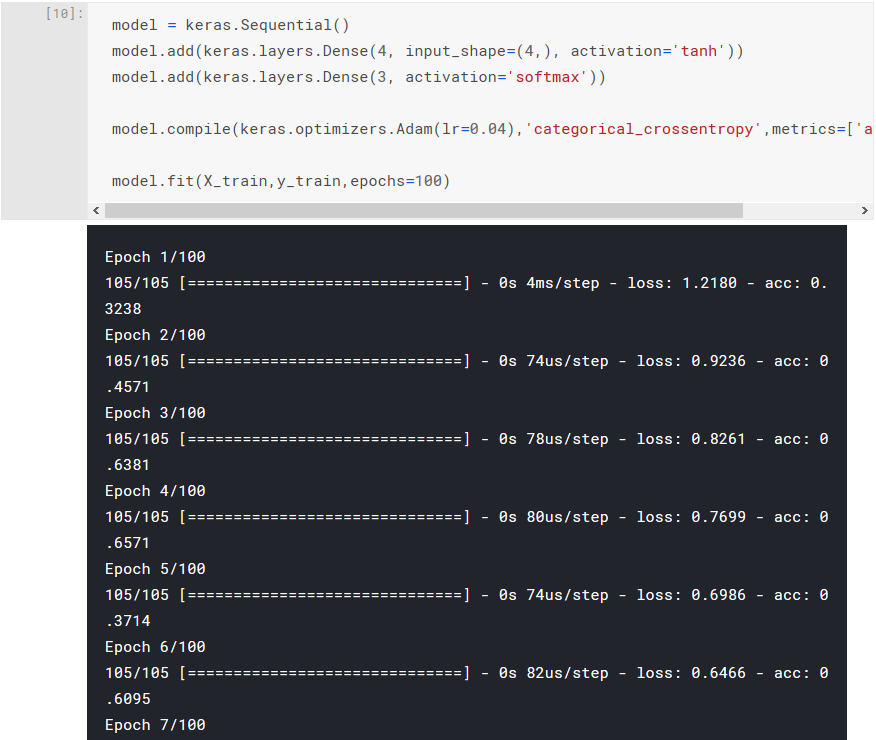### Testing the model

After the neural network has been trained, we want to evaluate it against our test set and output its accuracy. The evaluate() method returns a list containing the loss value at index 0 and in this case, the accuracy metric at index 1.

accuracy = model.evaluate(X_test, y_test)


If we run all the code up until this point, and we output the contents of our accuracy variable, we should see something similar to the following: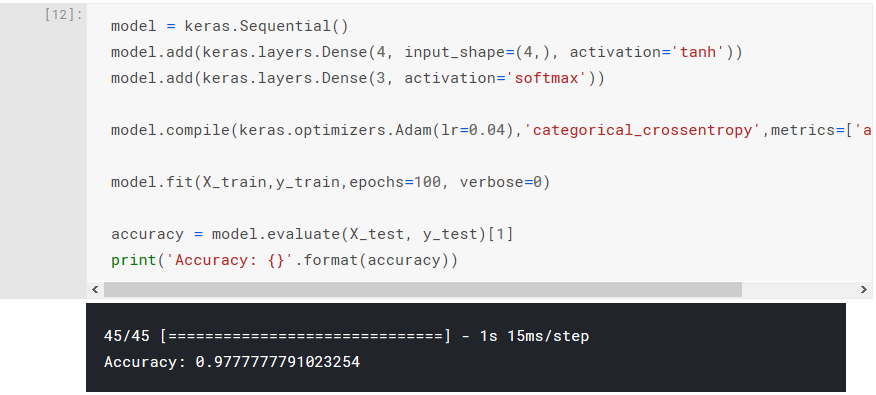### Generating all our results

Up until this point, we have successfully prepared the Iris Flower dataset, configured our model, trained our model, evaluated it using the test set, and reported its accuracy. However, this reported accuracy is only one sample of our desired thirty.

We can do this with a simple loop to repeat the process thirty times, and a list to store all the results. This only requires some minor modifications to our existing code:

results_control_accuracy = []
for i in range(0,30):
model = keras.Sequential()

model.fit(X_train,y_train,epochs=100, verbose=0)

accuracy = model.evaluate(X_test, y_test)
results_control_accuracy.append(accuracy)

print(results_control_accuracy)


This will take a few minutes to execute depending on whether you're using a Kaggle Kernel notebook or your own development environment, but once it has you should see a list containing the accuracy results for all thirty of the executions (but your results will vary):

[0.9333333359824286, 0.9777777791023254, 0.9777777791023254, 0.9777777791023254, 0.9777777791023254, 0.9777777791023254, 0.9777777791023254, 0.9777777791023254, 0.9555555568801032, 0.9777777791023254, 0.9777777791023254, 0.9777777791023254, 0.9777777791023254, 0.6000000052981906, 0.9777777791023254, 0.9777777791023254, 0.9777777791023254, 0.9111111124356588, 0.9777777791023254, 0.9777777791023254, 0.9777777791023254, 0.9777777791023254, 0.9777777791023254, 0.9777777791023254, 0.9555555568801032, 0.9777777791023254, 0.9777777791023254, 0.9777777791023254, 0.9777777791023254, 0.9111111124356588]


These are the results for our control arm, let's now do the same for our experimental arm. The experimental arm only has one difference: the number of neurons on the hidden layer. We can re-use our code for the control arm and just make a single modification where:

model.add(keras.layers.Dense(4, input_shape=(4,), activation='tanh'))


is changed to:

model.add(keras.layers.Dense(5, input_shape=(4,), activation='tanh'))


Of course, we'll also need to change the name of the list variable so that we don't overwrite the results for our control arm. The code will end up looking like this:

results_experimental_accuracy = []
for i in range(0,30):
model = keras.Sequential()

model.fit(X_train,y_train,epochs=100, verbose=0)

accuracy = model.evaluate(X_test, y_test)
results_experimental_accuracy.append(accuracy)

print(results_experimental_accuracy)


After executing the above and waiting a few minutes, we will have our second set of results:

[0.9111111124356588, 0.9555555568801032, 0.9555555568801032, 0.9777777791023254, 0.9777777791023254, 0.9777777791023254, 0.9555555568801032, 0.933333334657881, 0.9777777791023254, 0.9777777791023254, 0.9777777791023254, 0.9555555568801032, 0.9777777791023254, 0.933333334657881, 0.9777777791023254, 0.9777777791023254, 0.9777777791023254, 0.9777777791023254, 0.9777777791023254, 0.9777777791023254, 0.9777777791023254, 0.9777777791023254, 0.9333333359824286, 0.9777777791023254, 0.9777777791023254, 0.9333333359824286, 0.9777777791023254, 0.9555555568801032, 0.9777777791023254, 0.9777777791023254]


### Saving the results

The results for our experiment have been generated, and it's important that we save them somewhere, so we that can use them later. There are multiple approaches to saving or persisting your data, but we are going to make use of pandas.DataFrame.to_csv():

pd.DataFrame(results_control_accuracy).to_csv('results_control_accuracy.csv', index=False)

pd.DataFrame(results_experimental_accuracy).to_csv('results_experimental_accuracy.csv', index=False)


The above code will save your results to individual files corresponding to the arm of the experiment. Where the files go depend entirely on your development environment. If you're developing in your own local environment, then you will likely find the files in the same folder as your notebook or script. If you're using a Kaggle Notebook, it is important that you click the blue commit button in the top right of the page.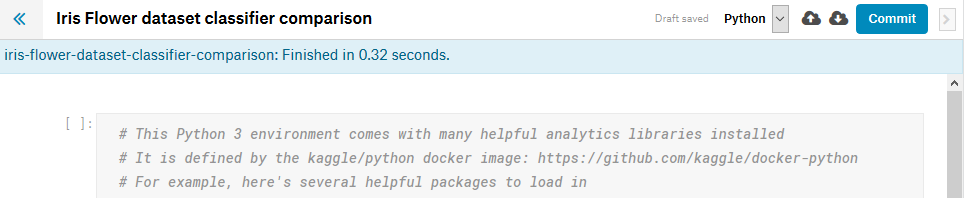It will take a few minutes to commit your notebook but once it's done, you know your file is safe. It's not immediately obvious where the files have been stored, but you can double check their existence by repeating the following steps: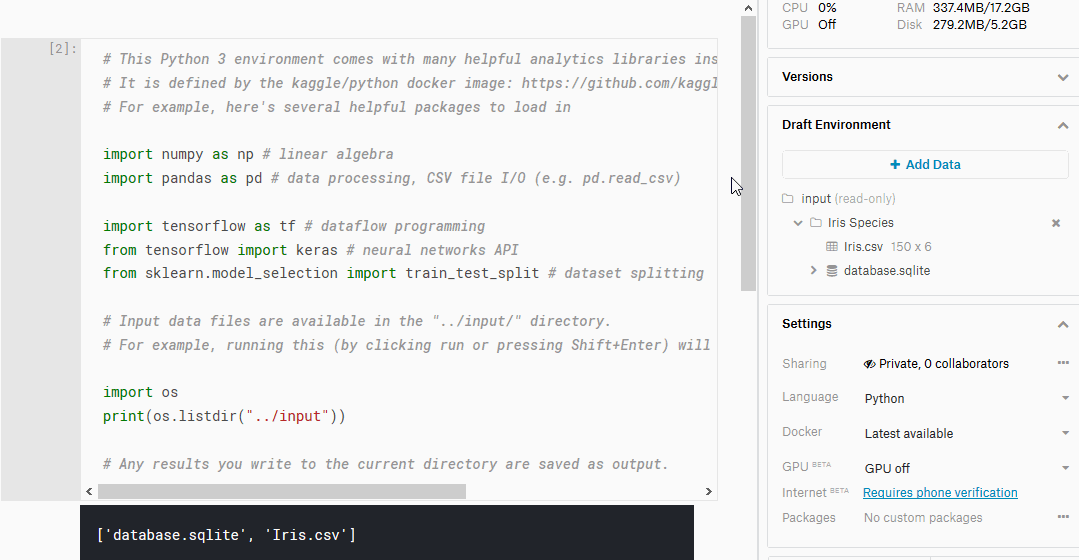## Conclusion

In this article we prepared our dataset such that it was ready to be fed into our neural network training and testing process. We then built and trained our neural network models using Python and Keras, followed by some simple automation to generate thirty samples per arm of our experiment.

In the next part of this four-part series, we will have a look at how our solutions performed and discuss anything interesting about the results. This will include some visualisation, and we may even return to our experiment code to produce some new results.